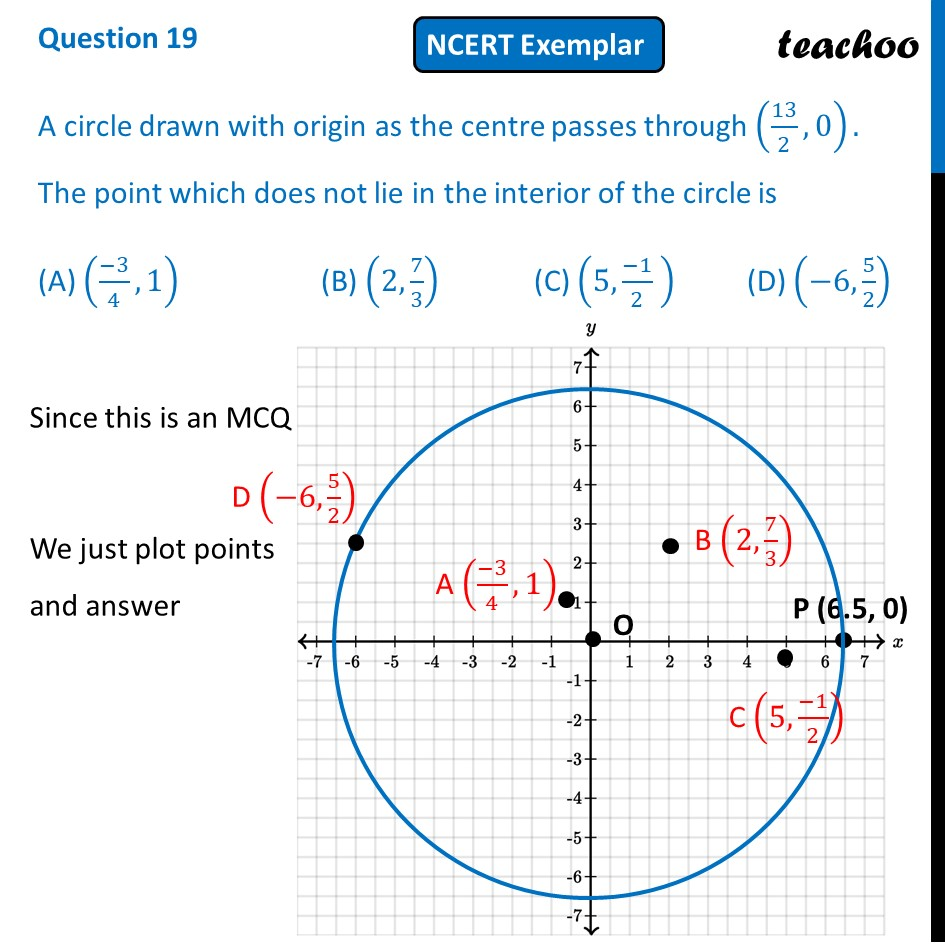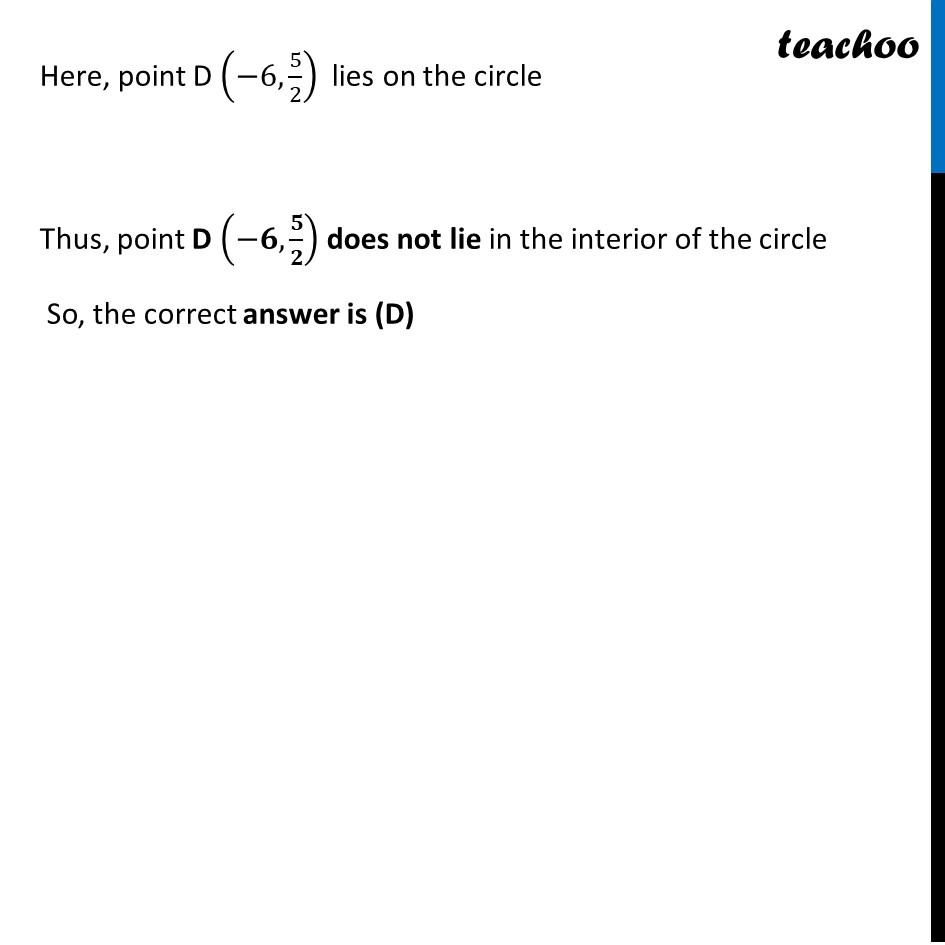NCERT Exemplar - MCQ

Chapter 7 Class 10 Coordinate Geometry
Serial order wise

## (A) ((-3)/4,1)    (B) (2, 7/3)       (C) (5,(-1)/2  )    (D) (-6, 5/2)Learn in your speed, with individual attention - Teachoo Maths 1-on-1 Class

### Transcript

Question 19 A circle drawn with origin as the centre passes through (13/2, 0). The point which does not lie in the interior of the circle is (A) ((−3)/4,1) (B) (2, 7/3) (C) (5,(−1)/2 ) (D) (−6, 5/2) Since this is an MCQ We just plot points and answer Here, point D (−6, 5/2) lies on the circle Thus, point D (−𝟔,𝟓/𝟐) does not lie in the interior of the circle So, the correct answer is (D)